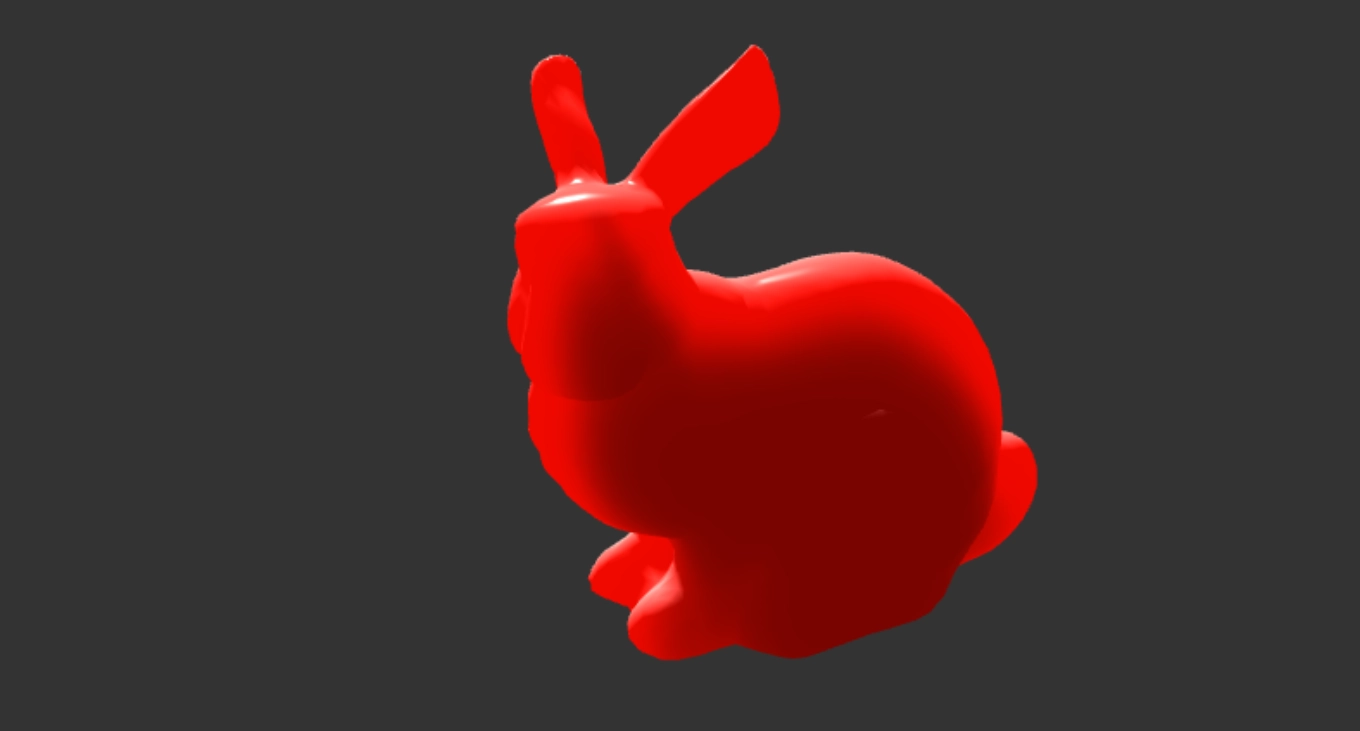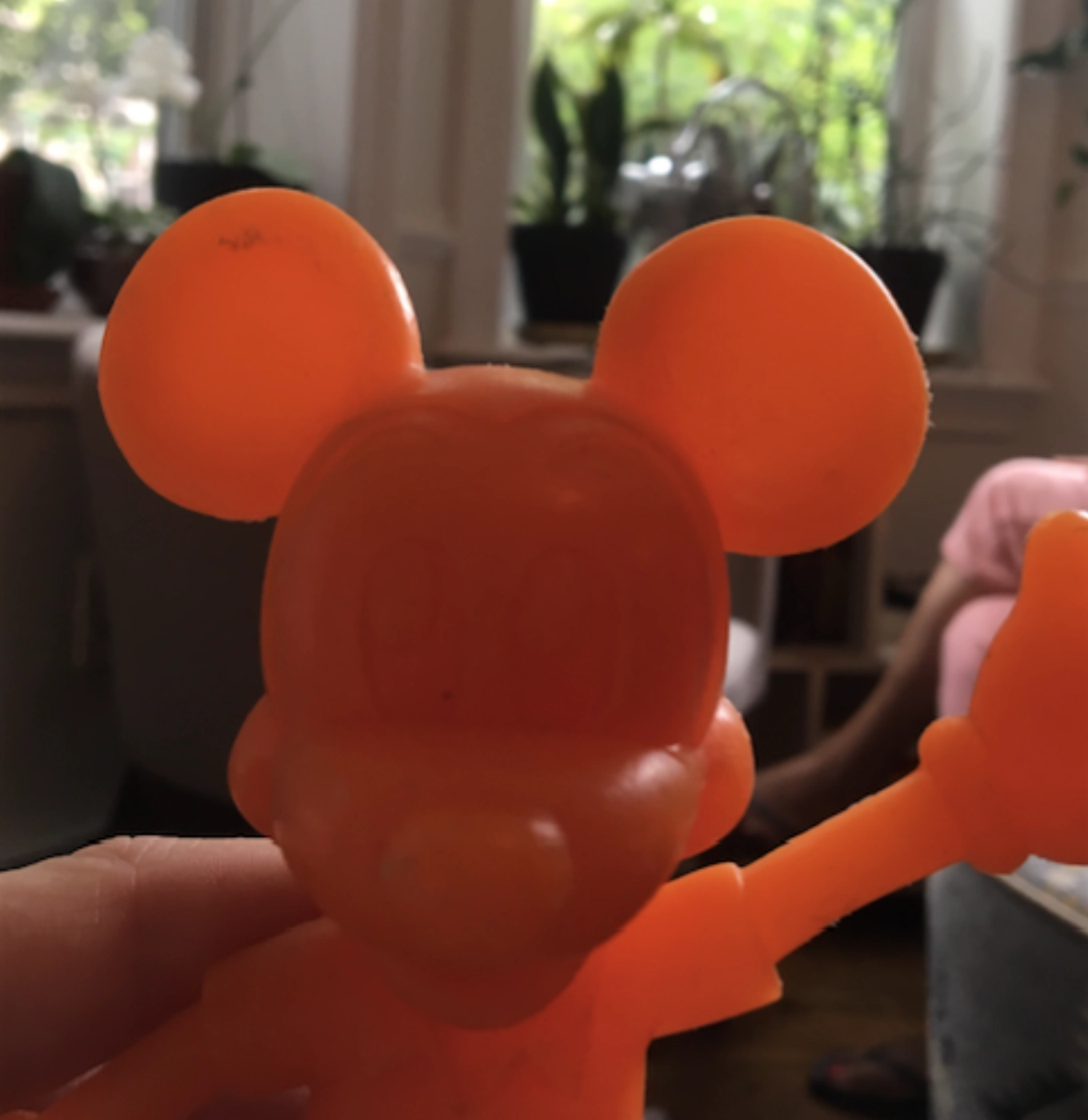# Approximating Subsurface Scattering With 3D Distance FieldsSubsurface scattering happens when photons penetrate a translucent surface and scatters within the material until they exit at a different location.A rendering technique that can be used to render subsurface scattering is a depth map approach. In this technique, a depth map is generated from the light point of view, similarly to a shadow mapping techniques. Then, the thickness of the model at a given fragment can be retrieved by sampling the depth map and comparing it to the current fragment depth. While this technique gives good results, choosing a depth bias can be difficult, and convex models might show artifacts.

As an experiment and alternative to the depth map based approach, here is a technique using a 3D distance field that once generated, can be used to sample the mesh thickness.

A 3D distance field is a representation in which, for each point of the extent of the space we work with, we associate a normalized distance to the mesh; where a positive distance means we are outside the mesh, and a negative distance means we are within.

When storing a 3D distance field in a texture, we remap the distance values within the range $[0..1]$, where anything in the range $[0..0.5[$ is outside the mesh, and $[0.5..1]$ is inside.

A small header only library I wrote to generate a 3D texture from a mesh is available here: sdf.h. It takes in a mesh and outputs a 3D distance field texture ready to be uploaded on the GPU:

unsigned int* sd_texture3d(sd_mesh_t const* mesh, int resx, int resy, int resz)


Here is a view of the different slices of the 3D texture distance field of the famous 3D model suzanne from Blender:

Then, when rendering the triangle mesh, each vertex is transformed in UV space.

vec3 ToUVSpace(vec3 position) {
return (position + abs(u_min)) / (u_max - u_min);
}


Where u_min, and u_max are the extent of the bounding box containing the 3D mesh, this remaps the position values to the values to [0..1].

The next step is to step through the texture cube starting from the current fragment UV. The direction used to step through the 3D texture cube is given by the view direction. For each step through the 3D texture along the ray, we accumulate the thickness by marching towards the light direction.

The distance field when sampled from the texture, as mentionned previously, has the following meaning:

• if sampledValue < 0.5: inside the triangle mesh
• if sampledValue > 0.5: outside the triangle mesh

Positive distances (outside the mesh) will have no contribution to the thickness while negative distance (inside the mesh) in the field will contribute:

sdf = texture(u_texture, uv).r;
sdf = (1.0 - step(0.5, sdf)) * sdf;
thicknessInLightDirection += sdf / steps;